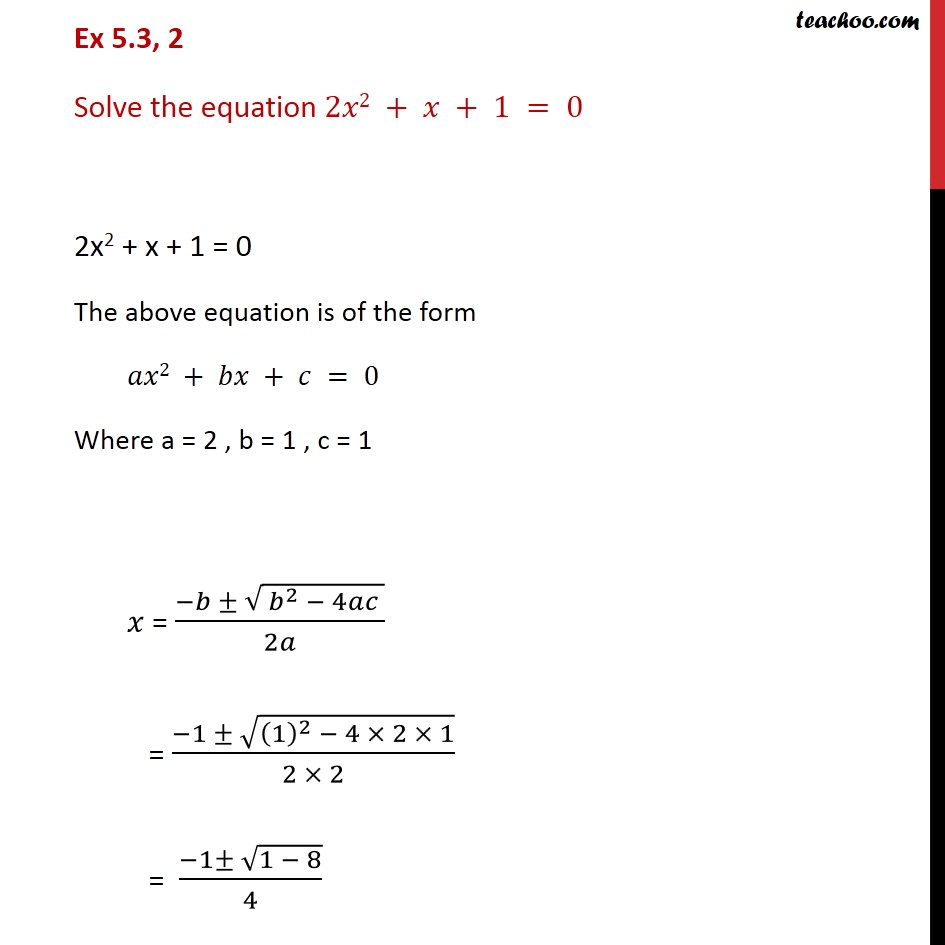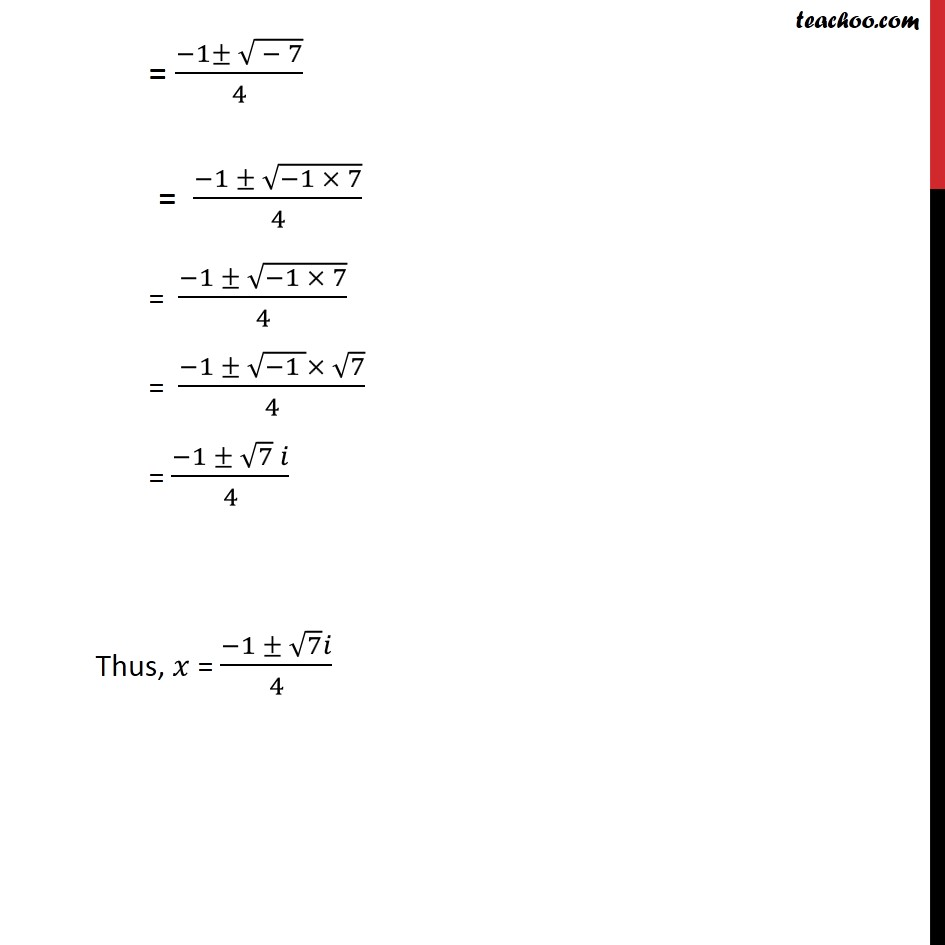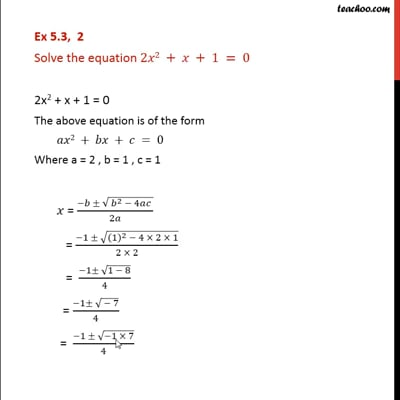Ex 5.3

Chapter 5 Class 11 Complex Numbers
Serial order wiseThis video is only available for Teachoo black users

Solve all your doubts with Teachoo Black (new monthly pack available now!)

### Transcript

Ex 5.3, 2 Solve the equation 2𝑥2 + 𝑥 + 1 = 0 2x2 + x + 1 = 0 The above equation is of the form 𝑎𝑥2 + 𝑏𝑥 + 𝑐 = 0 Where a = 2 , b = 1 , c = 1 𝑥 = (−𝑏 ± √( 𝑏^2 − 4𝑎𝑐 ))/2𝑎 = (−1 ± √((1)^2 − 4 × 2 × 1))/(2 × 2) = (−1± √(1 − 8))/4 = (−1± √( − 7))/4 = (−1 ± √(−1 × 7))/4 = (−1 ± √(−1 × 7))/4 = (−1 ± √(−1 )× √7)/4 = (−1 ± √7 𝑖)/4 Thus, 𝑥 = (−1 ± √7 𝑖)/2